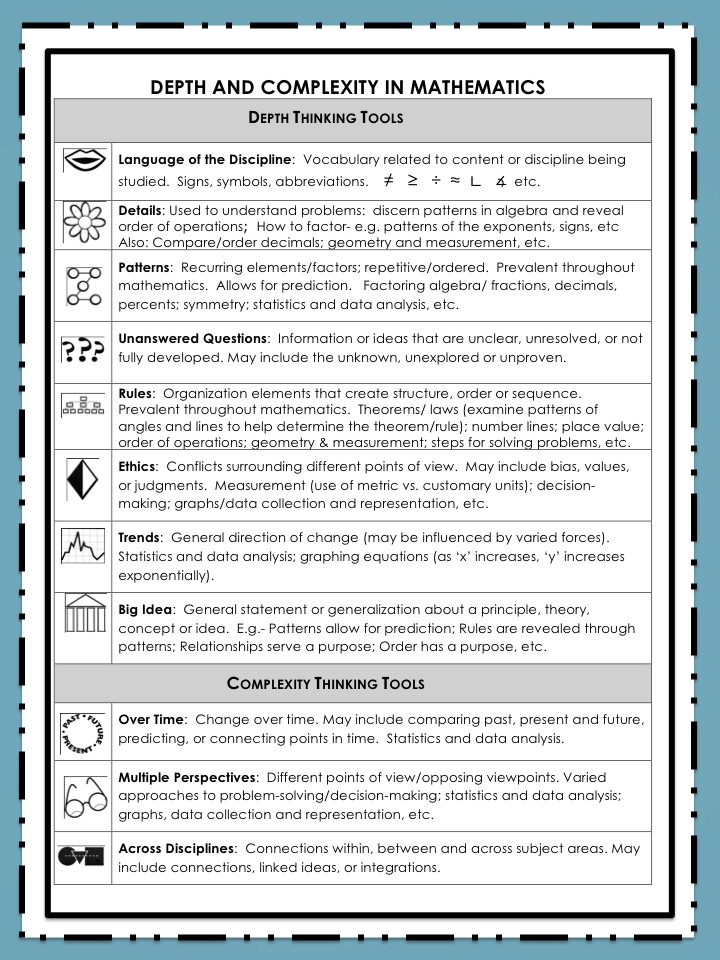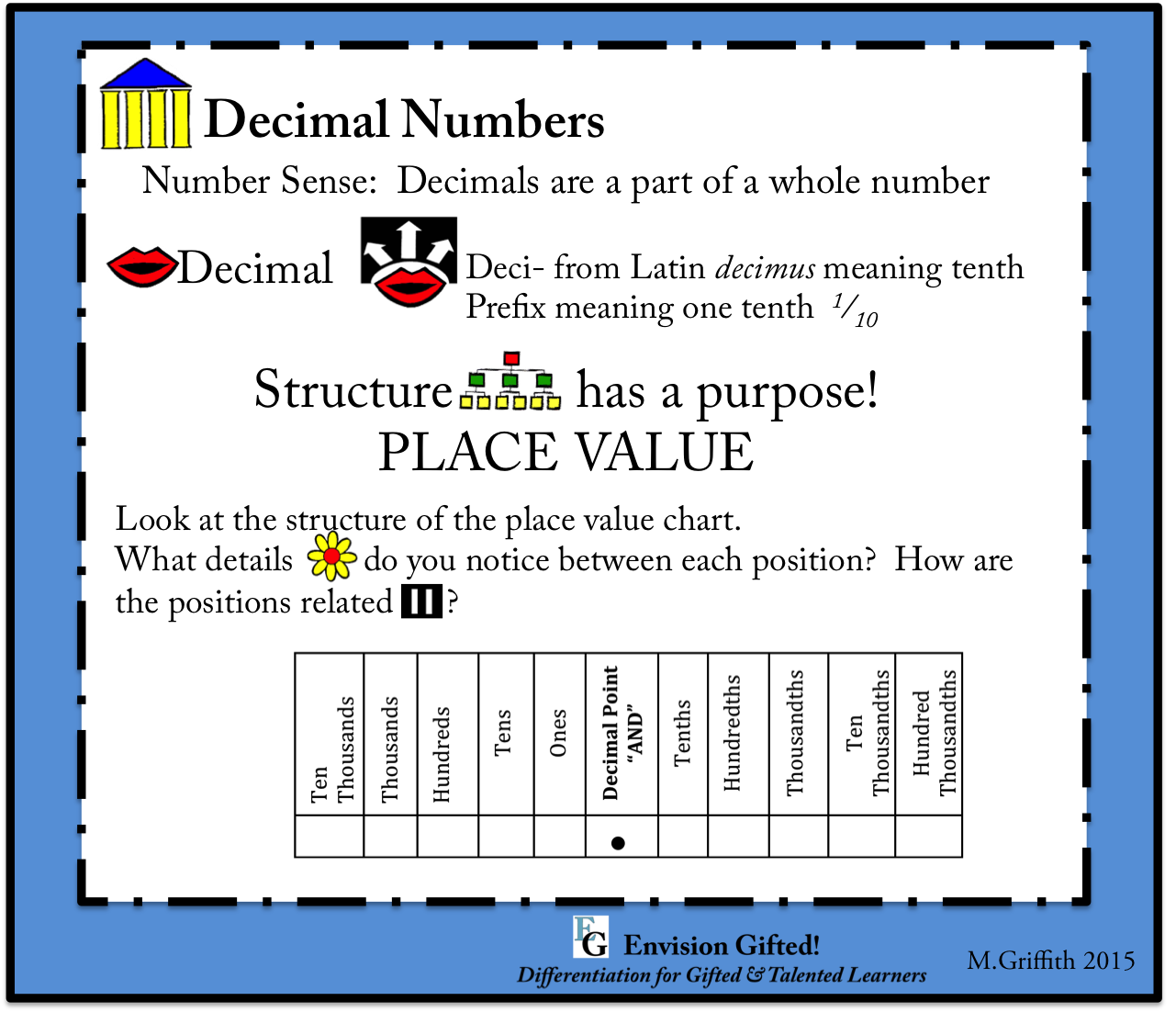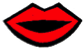# Depth and Complexity in Math

### How do the dimensions of depth and complexity fit into mathematics?Because the Depth and Complexity icons are tools that facilitate a more sophisticated understanding of a concept, they are not limited to a single content area.  These tools are a natural fit for developing a deeper understanding of mathematics.

Indeed, one cannot obtain mastery without being able to speak the language of math, or to understand the details of a number (or problem), or how a number (problem/or solution) is structured.

Consider the many ways these tools can be applied to math.

## Depth and Complexity in Math: Individual Icons

### Decimal NumbersUse the Depth and Complexity Framework to help students understand decimal numbers.  Often students rush to the operational manipulation of decimals without really understanding decimal numbers. This can leave them at a loss when asked to explain the concept, why they did what they did, or what their answer means.Start by introducing students to the language of the discipline.  What is a decimal number?  Lead them to the understanding by discussing the origin of the word.Deci- from Latin  decimus meaning “tenth”.  You can also point out to students that “Deci” is a prefix that means one-tenth (1/10). This understanding ties nicely to the generalization, “STRUCTURE HAS A PURPOSE” and focusing on place value.Students working in partners or small groups- Have them examine a place value chart and look for details in the structure of the chart.  How are the place value positions related?

Direct students to write a multiplication rule to help get from the ones position to the tens position that would also hold true for the tens to the hundreds position, and the hundreds to thousands position, etc.

Ask students if that same rule holds true when applied to the positions to the right of the decimal point?  Why/why not?

### Differentiate Math: Compare and Order DecimalsStudents work with decimals to rank times from the Women’s Luge in the the 2014 Winter Olympics.  They then answer questions based on their findings.